Tavish Srivastava — Published On December 1, 2013 and Last Modified On June 25th, 2020

My first analytics project involved predicting business from each sales agent and coming up with a targeted intervention for each agent.

I built my first linear regression model after devoting a good amount of time on data cleaning and variable preparation. Now was the time to access the predictive power of the model. I got a MAPE of 5%, Gini coefficient of 82% and a high R-square. Gini and MAPE are metrics to gauge the predictive power of linear regression model. Such Gini coefficient and MAPE for an insurance industry sales prediction are considered to be way better than average. To validate the overall prediction we found the aggregate business in an out of time sample. I was shocked to see that the total expected business was not even 80% of the actual business. With such high lift and concordant ratio, I failed to understand what was going wrong. I decided to read more on statistical details of the model. With a better understanding of the model, I started analyzing the model on different dimensions. After a close examination of residual plots, I found that one of the predictor variables had a square relationship with the output variable.Since then, I validate all the assumptions of the model even before reading the predictive power of the model. This article will take you through all the assumptions in a linear regression and how to validate assumptions and diagnose relationship using residual plots.

[stextbox id=”section”]Assumptions of Linear Regression Model :[/stextbox]

There are number of assumptions of a linear regression model. In modeling, we normally check for five of the assumptions. These are as follows :

1. Relationship between the outcomes and the predictors is linear.
2. Error term  has mean almost equal to zero for each value of outcome.
3. Error term  has constant variance.
4. Errors are uncorrelated.
5. Errors are normally distributed or we have an adequate sample size to rely on large sample theory.

The point to be noted here is that none of these assumptions can be validated by R-square chart, F-statistics or any other model accuracy plots. On the other hand, if any of the assumptions are violated, chances are high that accuracy plot can give misleading results.

[stextbox id=”section”]How to use residual for diagnostics :[/stextbox]

Residual analysis is usually done graphically. Following are the two category of graphs we normally look at:

1. Quantile plots : This type of is to assess whether the distribution of the residual is normal or not. The graph is between the actual distribution of residual quantiles and a perfectly normal distribution residuals. If the graph is perfectly overlaying on the diagonal, the residual is normally distributed. Following is an illustrative graph of approximate normally distributed residual.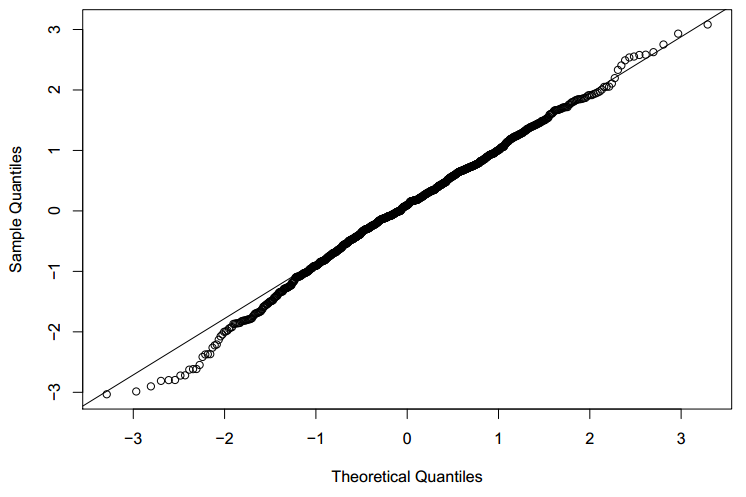Let’s try to visualize a quantile plot of a biased residual distribution.In the graph above, we see the assumption of the residual normal distribution being clearly violated.

2. Scatter plots:  This type of graph is used to assess model assumptions, such as constant variance and linearity, and to identify potential outliers. Following is a scatter plot of perfect residual distributionLet’s try to visualize a scatter plot of residual distribution which has unequal variance.In the graph above, we see the assumption of the residual normal distribution being clearly violated.

[stextbox id=”section”]Example :[/stextbox]

For simplicity, I have taken an example of single variable regression model to analyze residual curves. Similar kind of approach is followed for multi-variable as well.

Say, the actual relation of the predictor and the output variable is as follows: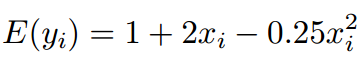Ignorant of the type of relationship, we start the analysis with the following equation.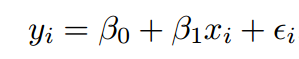Can we diagnose this misfit using residual curves?

After making a comprehensive model, we check all the diagnostic curves. Following is the Q-Q plot for the residual of the final linear equation.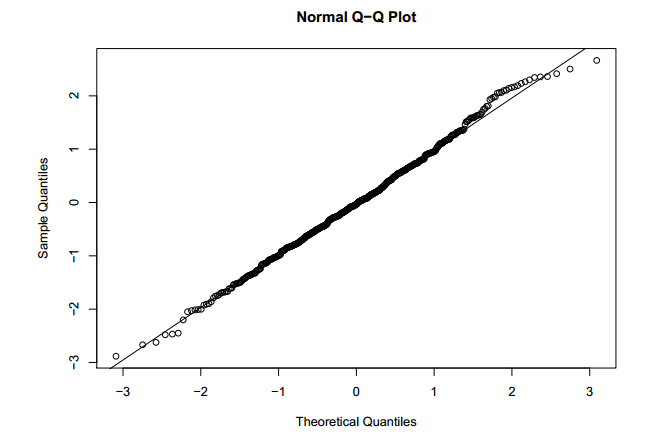Q-Q plot looks slightly deviated from the baseline, but on both the sides of the baseline. This indicated residuals are distributed approximately in a normal fashion.

Following is the scatter plot of the residual :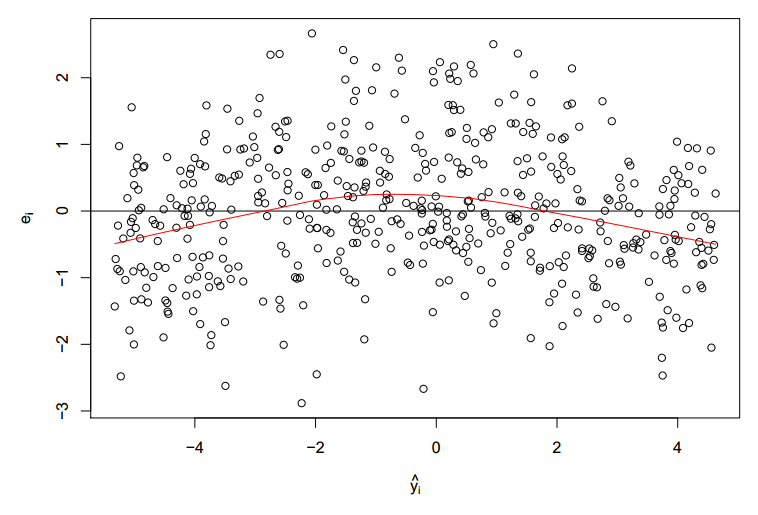Clearly, we see the mean of residual not restricting its value at zero. We also see a parabolic trend of the residual mean. This indicates the predictor variable is also present in squared form. Now, let’s modify the initial equation to  the following equation :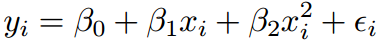Following is the new scatter plot for the residual of the new equation :We now clearly see a random distribution and a approximate zero residual mean.

[stextbox id=”section”]End Notes:[/stextbox]

Every linear regression model should be validated on all the residual plots . Such regression plots directionaly guides us to the right form of equations to start with. You might also be interested in the previous article on regression ( https://www.analyticsvidhya.com/blog/2013/10/trick-enhance-power-regression-model-2/ )

Do you think this provides a solution to any problem you face? Are there any other techniques you use to detect the right form of relationship between predictor and output variables ? Do let us know your thoughts in the comments below.

## If you like what you just read & want to continue your analytics learning, subscribe to our emails or like our facebook page.###### Tavish Srivastava

Tavish Srivastava, co-founder and Chief Strategy Officer of Analytics Vidhya, is an IIT Madras graduate and a passionate data-science professional with 8+ years of diverse experience in markets including the US, India and Singapore, domains including Digital Acquisitions, Customer Servicing and Customer Management, and industry including Retail Banking, Credit Cards and Insurance. He is fascinated by the idea of artificial intelligence inspired by human intelligence and enjoys every discussion, theory or even movie related to this idea.

## 9 thoughts on "Diagnosing residual plots in linear regression models"###### Tuhin Chattopadhyay says:December 02, 2013 at 11:37 am
Good one Kunal, though I prefer to use designated tests to verify each of the assumptions. Reply###### Tuhin Chattopadhyay says:December 02, 2013 at 11:39 am
Sorry, it's by Tavish. Liked it Tavish. Reply###### Tavish Srivastava says:December 02, 2013 at 11:45 am
Tuhin, I agree doing designated test to verify each assumption is a more sturdy approach. However, using the approach mentioned in the article will tell you two facts. First, whether assumption is validated or not. Second, if assumptions are not validated, what will be the best starting point for the next iteration. For instance, in the example mentioned in the article, residual plot gave us a better starting equation. Please share your thoughts of how will designated tests help on the second point. Thanks, Tavish Reply###### Kunal Jain says:December 02, 2013 at 4:38 pm
Tuhin, While the designated tests would provide you the output, they do not provide as much details about why things have failed. Also, even if you use designated tests, it makes sense to understand what has failed. Thanks, Kunal Reply###### Tuhin Chattopadhyay says:December 03, 2013 at 6:09 am
Dear Kunal and Tavish, Both the presumptive and confirmatory tests are required in any field of science. It's not about a fight of graphical method vs. the tests. It's about performing both the things sequentially. For instance, you can have a look of the distribution (normal) graphically at first by histogram of residuals or normal probability plot (NPP) and then apply Anderson Darling test in Minitab or Jarque Bera test in E-Views or Kolmogorov-Smirnov test and Shapiro Wilk test in SPSS to confirm. That's how I perform it. Reply###### Kunal Jain says:December 05, 2013 at 5:04 pm